Two-dimensional channel flow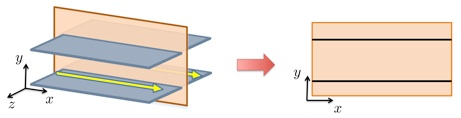Geometry of a two-dimensional channel flow.

The Orr-Sommerfeld equation governs the dynamics of two-dimensional velocity fluctuations around the laminar channel flow,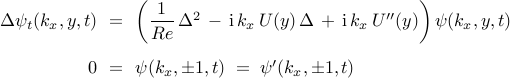where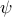— streamfunction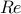— Reynolds number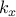— streamwise wavenumber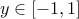— wall-normal coordinate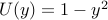— base flow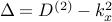— Laplacian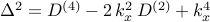— ‘‘square’’ of the Laplacian

For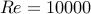(based on the centerline velocity and the channel half-width), the discretized version of the Orr-Sommerfeld equation is obtained using the pseudo-spectral scheme with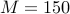colocation points in the wall-normal direction. Flow fluctuations with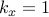are then advanced in time using the matrix exponential with time step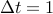and randomly generated initial profile (that satisfies both homogeneous Dirichlet and Neumann boundary conditions). After a transient period of ten time-steps,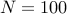snapshots are taken to form the snapshot matrices and apply the standard DMD algorithm along with its sparsity-promoting variant.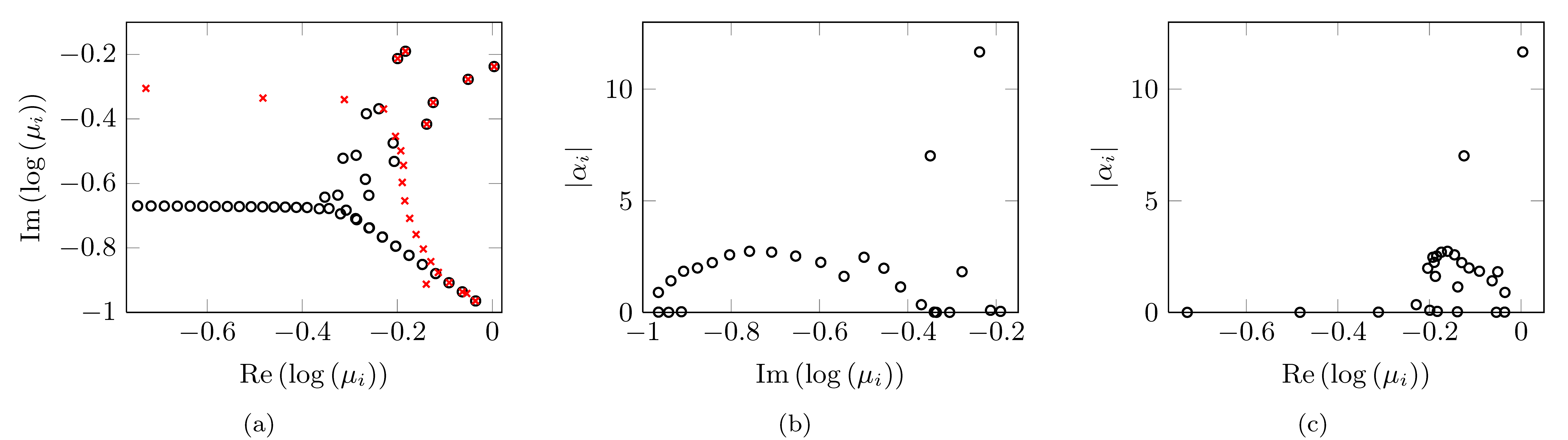(a) Spectrum of the Orr-Sommerfeld operator (circles) along with the eigenvalues resulting from the standard DMD algorithm (crosses) for the 2D channel flow withand. Dependence of the absolute value of the DMD amplitudes on (b) the frequency and (c) the real part of the corresponding DMD eigenvalues.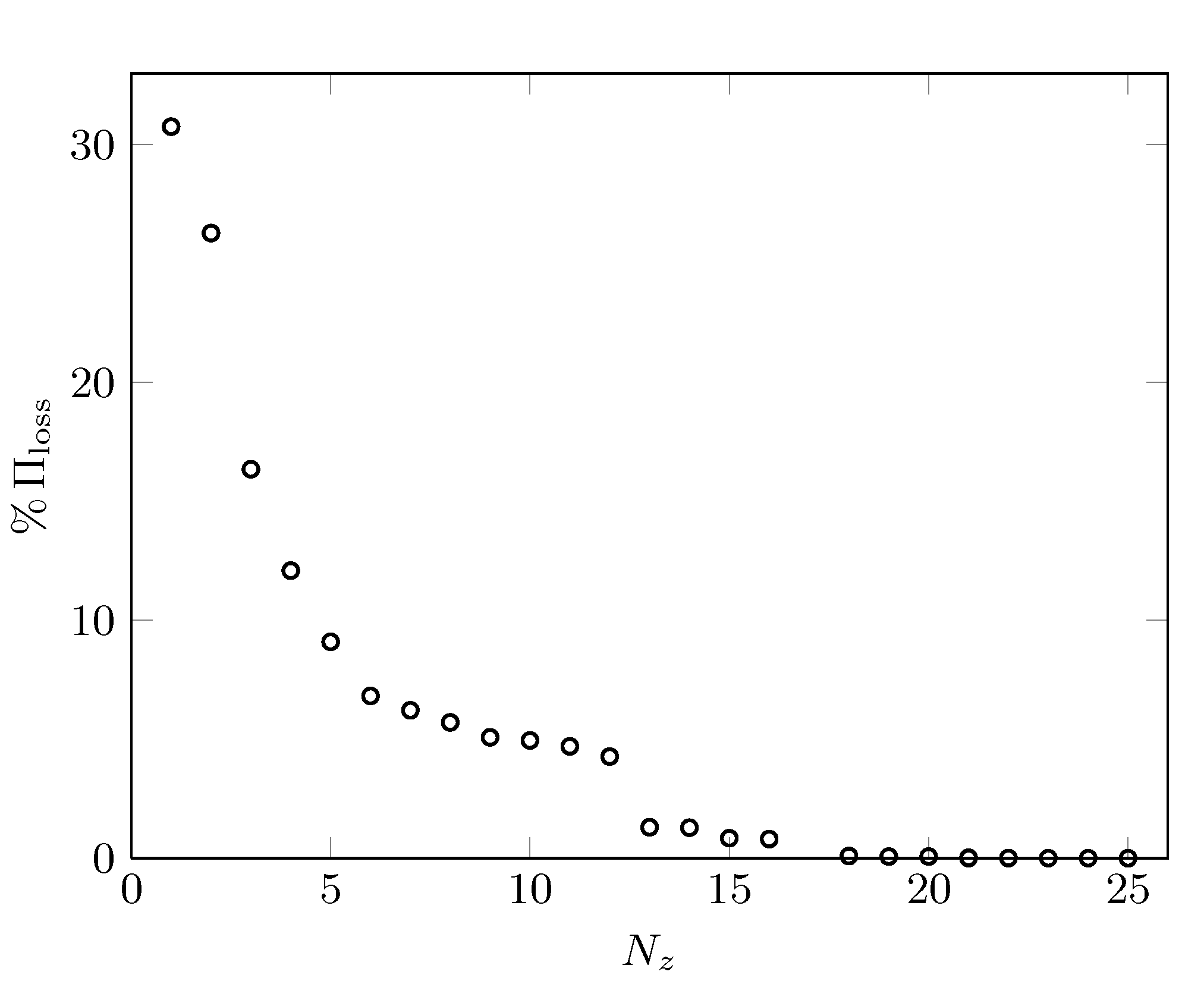Performance loss,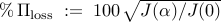, of the optimal vector of amplitudes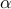resulting from the sparsity-promoting DMD algorithm with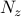DMD modes.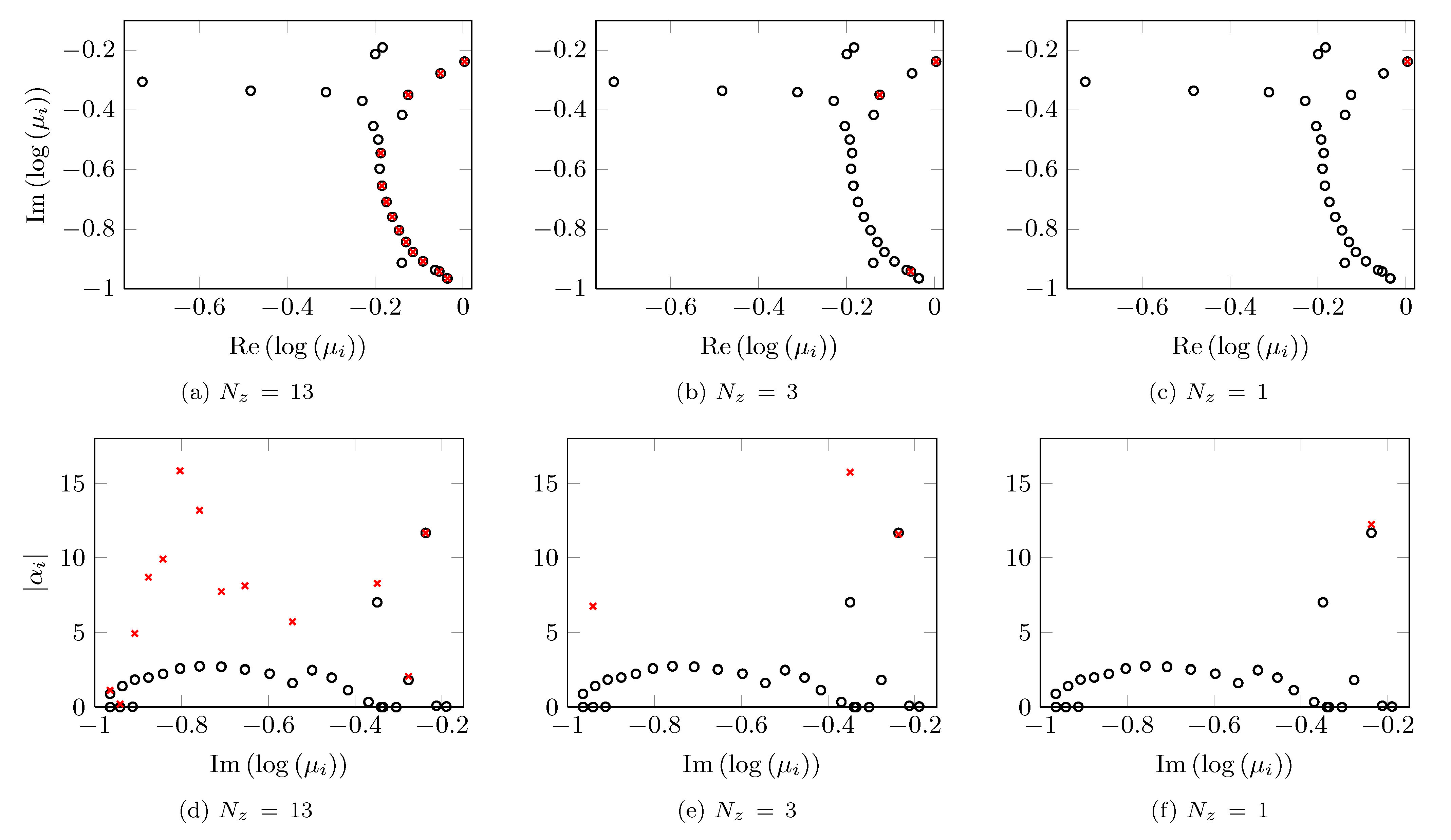Eigenvalues of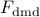(first row) and the absolute values of the DMD amplitudes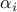(second row). The results are obtained using the standard DMD algorithm (circles) and the sparsity-promoting DMD algorithm (crosses) withDMD modes.